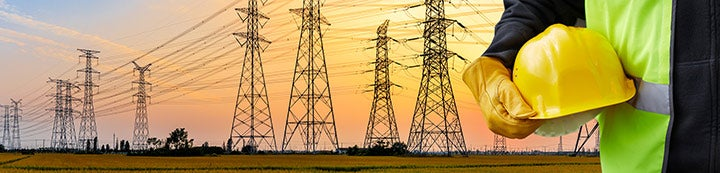1 - 9 of 9 Posts

Jmp2016

·
Registered
Joined
·
2 Posts
Discussion Starter · ·
3 phase 4 wire 120/208 system. Phase A pulling 50 amps, phase B pulling 50 amps, phase C pulling 0 amps. Is the neutral carrying a load? Or is does the system balance and leave no load on the neutral. OR would phase C need to pull 50 amps as well to fully balance the system.

Possibly the same question but maybe not...
3 phase 4 wire 120/208 system. Circuit 1, 3 and 5 (first three positions on the left side of the panel) have a 3 pole 20 breaker in place and a 3 phase network circuit going out with a shared neutral. Circuit 1 powers a row of lights (5 amps), circuit 3 powers a row of lights (5 amps) and circuit 5 doesn't power anything. Does the branch circuit shared neutral balance and carry 0 or would it need circuit 5 to also carry 5 amps.

I know in a single phase scenario both cases would result in a balanced load because the two phases are perfectly out of phase with each other by 180 degrees, but with three phase they are out of phase with each other by 120 degrees each which is why I could see the above scenarios balancing to some degree but still leaving some unbalanced load on the neutral- unless all 3 were perfectly equal. Thoughts?

emtnut

·
Band Member
DIYer Extrodinaire
Joined
·
8,262 Posts
3 phase 4 wire 120/208 system. Phase A pulling 50 amps, phase B pulling 50 amps, phase C pulling 0 amps. Is the neutral carrying a load? Or is does the system balance and leave no load on the neutral. OR would phase C need to pull 50 amps as well to fully balance the system.

The system is looked at as vectors, all 3 120˚ apart.

since phase C is zero, the neutral current is sin120˚ X 50 A = 43.3

•glen1971, lighterup and Max C.

brian john

·
Registered
Joined
·
32,124 Posts
Simple formula to remember for 3 Phase Neutral Current.

If A, B and C are the three phase currents, the formula to find the neutral current is the square root of the following:

(A*A + B*B+C*C) - (A*B + A*C + B*C).

I have a better looking formula at work but for now.

•glen1971 and frenchelectrican

Jmp2016

·
Registered
Joined
·
2 Posts
Discussion Starter · ·
Ok thanks guys so following that formula..
It simplifies to sqrt ((50^2+50^2+0^2)-(50 x 50 + 50 x 0 + 50 x 0))
Sqrt (5000-2500)
Sqrt (2500)
=50. So would the unbalanced load on the neutral be 50 or am I missing something and it's 43?

Thanks,
Joe

dmxtothemax

·
Registered
Joined
·
2,969 Posts
Your missing the point that the phases are 120 degee's apart not 180 !
That's why its 43A not 50A.
study how phase angles effect voltages !

telsa

·
Registered
Joined
·
14,469 Posts
As field electricians, such EE calculations are the stuff of apprentices -- and the occasional j-man test.

We build to the worst case -- ie fattest amps.

In a 208Y120 or 480Y277 system -- one just does not down size neutrals.

Whereas it's common for the Poco to reduce its own Service Laterals to such Services.

The Poco is counting on some load balance -- we can't ever.

As for homework// test questions -- they are answered -- virtually without limit -- in the back threads here at ET.

·
Joined
·
12,636 Posts

emtnut

·
Band Member
DIYer Extrodinaire
Joined
·
8,262 Posts
I would have thought 50 just visualizing the triangle. You have two equal sides at an angle of 60* and a third side of zero length. The neutral current is the length of the segment to close off the triangle.

http://www.jirvine.co.uk/Electrical_ Principles/Tutorial_13_3_Phase_AC/Images/Phas_02.gif

If the other two phases are 50 each, what would you need to balance this on the third phase? It's 50 right?
Yes, you're right :thumbsup:

I was thinking (well, I don't know WHAT I was thinking) ... about the x axis magnitude (@ 120˚)

The neutral current would be 50A

Thanks for catching that Splatzy :thumbsup:

•hardworkingstiff and splatz

RePhase277

·
Banned
Joined
·
11,885 Posts
As field electricians, such EE calculations are the stuff of apprentices -- and the occasional j-man test.

We build to the worst case -- ie fattest amps.

In a 208Y120 or 480Y277 system -- one just does not down size neutrals.

Whereas it's common for the Poco to reduce its own Service Laterals to such Services.

The Poco is counting on some load balance -- we can't ever.

As for homework// test questions -- they are answered -- virtually without limit -- in the back threads here at ET.
You are a bigot.... you didn't even mention. 600Y/347. There's a new Canuckian here every day!

•glen1971
1 - 9 of 9 Posts